require(nycflights13)
require(tidyverse)

knitr::opts_chunk$set( cache = TRUE, message = FALSE ) # 1 Working with R ## 1.1 Vectors purrr provides is_* functions for testing type (for logical, integer, double, numeric, character, atomic, list, vector). Vectors can be named on creation, like c(x = 1, y = 2, z = 3), or after the fact using purrr::set_names(1:3, c("a", "b", "c")). ### 1.1.1 Subsetting vectors When subsetting vectors, negative values drop elements at a specified position: x <- c(10, 11, 12, 13, 14, 15) x[-2] ##  10 12 13 14 15 x[c(-2, -3)] ##  10 13 14 15 # x[c(2, -3)] # can't be combined, returns error ### 1.1.2 Attributes Can be set using attr(): attr(x, "greeting") ## NULL attr(x, "greeting") <- "Hi!" attributes(x) ##$greeting
##  "Hi!"

## 1.2 Probability distributions

In R, every distribution has four functions, all of which have the syntax is letter + name of distribution

• d: finds the density for a certain value x (the density function)
• p: finds the cumulative probability given a quantile q (i.e., the CDF)
• q: finds the quantile given a cumulative probability p (i.e. the inverse CDF)
• r: generates n random values

The first argument of each function is either x, q, p, or n, and remaining arguments parametrize the distribution:

• runif(n, min = 0, max = 1)
• rbinom(n, size, p) where $$\text{Binom}(n, p)$$ corresponds to $$\text{Binom}(\text{size}, p)$$
• rt(n, df, ncp) where ncp is an optional non-centrality parameter
• rnorm(n, mean = 0, sd = 1)
• rexp(n, rate = 1)

and so forth for Beta (rbeta), Cauchy (rcauchy), Chi-Square (rchisq), F (rf), Gamma (rgamma), Geometric (rgeom), Hypergeometric (rhyper), Logistic (rlogis), Negative Binomial (rnbinom), Poisson (rpois), Studentized t (rtukey), Weibull (rweibull), and some others.

## 1.3 Iteration

### 1.3.1 General tips on iteration

#### 1.3.1.1 Modifying dataframes

Iterate along seq_along(df) or 1:ncol(df) or seq(ncol(df))(all are identical):

rand <- data.frame(matrix(rnorm(100, 0, 1), ncol = 10))

seq_along(rand)
##    1  2  3  4  5  6  7  8  9 10
seq(ncol(rand))
##    1  2  3  4  5  6  7  8  9 10
1:ncol(rand)
##    1  2  3  4  5  6  7  8  9 10
for(i in seq_along(rand)) {
rand[[i]] <- rand[[i]] * 10
}

#### 1.3.1.2 Storing loop-generated values

Always create the destination vector (or list) first and then filling up the vector, rather then expanding the vector (or dataframe, or string) on each loop iteration. Examples:

• To create a long string, create a list of strings, then combine at the end using one paste() call
• To generate a big dataframe row-wise, create a list of rows, then using dplyr::bind_rows() instead of rbind() in each loop iteration

### 1.3.3 Map family of functions from purrr

The map functions are similar to the apply functions, but provide a more consistent interface to iterate across a vector or list:

• map() returns a list, and is essentially identical to lapply()
• map_lgl() returns a logical (vector)
• map_int() returns an integer (vector)
• map_dbl() returns a double (vector)
• map_chr() returns a character (vector)
require(purrr)
map_dbl(rand, mean)
##         X1         X2         X3         X4         X5         X6
##  1.9180649 -1.8027837  0.3534937 -6.8938235  2.8662889  3.1523431
##         X7         X8         X9        X10
## -3.3233261 -2.4402544 -1.1798192 -1.1415138
map_dbl(rand, mean, trim = 0.5) # can pass additional arguments
##        X1        X2        X3        X4        X5        X6        X7
##  2.181209 -1.739250 -1.287261 -6.801780  3.120486  0.923228 -2.721336
##        X8        X9       X10
## -4.101704 -2.229284 -1.264049
rand %>% map_dbl(sd) # can use with pipe
##        X1        X2        X3        X4        X5        X6        X7
## 10.767827 13.404025 13.173023 12.256388 13.546651 11.994249  8.388493
##        X8        X9       X10
##  8.292800  9.676282  7.484229

Tools for working with several sub-categories, in order to fit models:

# verbose method
models <- mtcars %>%
split(.$cyl) %>% # equivalent to split(mtcars$cyl)
map(function(df) lm(mpg ~ wt, data = df)) # using anonymous function

# using purrr shortcuts
models <- mtcars %>%
split(.$cyl) %>% map(~lm(mpg ~ wt, data = .)) # now use map functinos models %>% map(summary) %>% map_dbl("r.squared") # equivalent to map_dbl(.$r.squared)
##         4         6         8
## 0.5086326 0.4645102 0.4229655

To iterate along two or more vectors, use map2() and pmap():

mu <- c(1, 5, 10)
sigma <- c(1, 0.1, 0.01)
n <- c(5, 6, 7)

map2(mu, sigma, rnorm, n = 5) # apply rnorm, first using different values of mu, then sigma
## []
##  0.7869046 1.5298229 1.8065202 0.9393476 0.4519518
##
## []
##  5.015145 5.000019 5.128891 4.993876 4.931637
##
## []
##   9.992750 10.027761  9.993880  9.997804 10.008609
map2(mu, sigma, rnorm, n = 5) %>% map(sum) # map2() returned a list, so we could use map() again
## []
##  4.637258
##
## []
##  24.96279
##
## []
##  49.99408
args1 <- list(mu, sigma, n)
args2 <- list(mean = mu, sd = sigma, n = n)
args3 <- data.frame(args2)

# by default, pmap does positional matching for function parameters
# here, we get a bad result because mu is listed first in args1, but rnorm takes n first
args1 %>% pmap(rnorm)
## []
##  -0.9153081
##
## []
##  10.842136  1.558008  8.593264 11.190593 -3.875410
##
## []
##     4.42207583   1.50713219   4.70768691 -13.63213417   8.13799988
##     1.50883349   0.03286155 -10.36769957  -1.31905532   0.66189064
# instead, pass a named list
args2 %>% pmap(rnorm)
## []
##   1.5394571  0.7347620  1.0581277  2.9806145 -0.1988132
##
## []
##  4.968141 4.993620 4.907933 5.002487 4.993384 4.828735
##
## []
##   9.990790 10.016362  9.969783 10.008348 10.004564 10.000355 10.013753
# pmap can also take a dataframe
args3 %>% pmap(rnorm) # the same thing
## []
##  0.8751826 2.3357094 2.3427401 0.7718666 1.2370848
##
## []
##  5.082546 4.962414 4.980114 4.953733 5.065688 4.829931
##
## []
##  10.008524 10.010003  9.996549 10.012196 10.003213 10.000935  9.998316

## 1.4 Logical operators

### 1.4.1 Comparison operators

• || and && are the standard logical operators
• | and & are vectorized comparison operators
• == is both a logical operator and vectorized
• To test for equality with NA, use is.na(x) rather than x == NA

### 1.4.2 Testing equality

Since R uses floating-point arithmetic, using == to test for equality might not always produce the desired results. dplyr::near(x, y) includes a built-in tolerance to safely compare values.

### 1.4.3 De Morgan’s law

As in any language:

• !(x & y) is equivalent to !x | !y
• !(x | y) is equivalent to !x & !y

## 1.6 The magrittr pipe

The pipe (%>%) feeds each previous output as the first argument of the next function.

require(dplyr)

trees %>%
group_by(borough) %>%
summarize(n = n(),
avg = mean(tree_dbh))
## # A tibble: 5 x 3
##   borough           n   avg
##   <fct>         <int> <dbl>
## 1 Bronx          1280  9.46
## 2 Brooklyn       2465 11.9
## 3 Manhattan       989  8.50
## 4 Queens         3679 12.7
## 5 Staten Island  1587 10.7
# SAME AS THIS (intermediates): gets tedious
# trees_grouped <- group_by(trees, borough)
# trees_summarized <- summarize(trees_grouped, n = n(), avg = mean(tree_dbh))

# SAME AS THIS (nesting): gets hard to read with other options and arguments
# trees_summarized <- summarize(group_by(trees, borough),
#                               n = n(), avg = mean(tree_dbh))

### 1.6.1 Variants of the pipe operator

• The tee pipe (%T>%) essentially “cuts” off a pipeline, returning the left hand side and simplying carrying out the actions on the right side
• The exposition pipe (%$%) explodes out (exposes) the variables of a dataframe to use in non-dataframe-based functions (such as functions in base-R) • The assignment pipe (%<>%) returns the entire pipeline and assigns it to the first variable (but use with caution, because can be unclear) ### 1.6.2 The attribute placeholder Using . tells R to use the previous passed value in a position that’s not the first position, unless . is used in a way where it’s nested, in which case it will still apply the first-argument rule: • f(y, z = x) is equivalent to x %>% f(y, z = .) • f(x, y = nrow(x), z = ncol(x)) is equivalent to x %>% f(y = nrow(.), z = ncol(.) • f(y = nrow(x), z = ncol(x) is equivalent to x %>% {f(y = nrow(.), z = ncol(.))} ## 1.7 R Markdown ### 1.7.1knitr chunk options Option Run code Show code Output Plots Messages Warnings eval = FALSE - - - - - include = FALSE - - - - - echo = FALSE - results = "hide" - fig.show = "hide" - message = FALSE - warning = FALSE - Syntax for setting global otions: knitr::opts_chunk$set(
message = FALSE,
fig.height = 4
)

### 1.7.2kable and kableExtra

knitr::kable() prints a prettier table:

require(knitr)
require(kableExtra)

seq <- data.frame(matrix(c(1, 2, 3, 4, 5, 6, 7, 8, 9), nrow = 3))
kable(seq, caption = "Sequential numbers") # works fine for PDF knitting, not pretty for HTML
Sequential numbers
X1 X2 X3
1 4 7
2 5 8
3 6 9
seq %>%
knitr::kable(caption = "Sequential numbers") %>%
kableExtra::kable_styling() # can be piped
Sequential numbers
X1 X2 X3
1 4 7
2 5 8
3 6 9

# 2 Data exploration and manipulation

## 2.1 Reading data using readr

Compared to reading data with base R, readr:

• Is faster
• Doesn’t convert strings to factors
• Handles dates better
• Shows a progress bar

General syntax:

read_csv(file,
col_names = TRUE, # set FALSE to treat first row as data
# set to vector to use custom colnames
col_types = NULL, # see below
skip = 0, # skip the first n lines
n_max = Inf, # only read up to n lines
na = c("", "NA")) # replace with NA

Other useful functions: read_lines(file) reads each line into a string

### 2.1.1 Datatype parsers in readr

Since readr doesn’t automatically convert characters to factors, we can set default datatypes using col_types:

read_csv("cleaned_subway_stations.csv",
col_types = cols(
.default = col_guess(),
CensusTract = col_factor()
))

Common parsers include:

• col_guess() is the default
• col_character(), col_double(), col_integer(), col_logical()
• col_datetime(format = ""), col_date(format = ""), col_time(format = "")
• col_factor(levels, ordered = FALSE), col_skip()

### 2.1.2 Reading other file types using haven

haven (also comes with the tidyverse) contains functions to read and write to other common formats. Specifically:

• Stata: read_dta(), which reads files up to version 15
• SAS: read_sas() reads .sas7bdat and .sas7bcat
• SPSS: read_sav() reads .sav

## 2.2 Aggregate statistics

• base::summary() provides the five-number summary, plus mean, in a named vector
• base::fivenum() provides the five-number summary in a non-named vector

### 2.2.1 Using by()

#s: by(values_to_aggregate, a_factor, function)
#r: always returns formatted output
by(trees$tree_dbh, trees$status, mean)
## trees$status: Alive ##  11.78739 ## -------------------------------------------------------- ## trees$status: Dead
##  5.36
## --------------------------------------------------------
## trees$status: Stump ##  0 by(trees[, c("tree_dbh", "latitude")], trees$status, summary)
## trees$status: Alive ## tree_dbh latitude ## Min. : 0.00 Min. :40.50 ## 1st Qu.: 5.00 1st Qu.:40.63 ## Median :10.00 Median :40.70 ## Mean :11.79 Mean :40.70 ## 3rd Qu.:17.00 3rd Qu.:40.76 ## Max. :79.00 Max. :40.91 ## -------------------------------------------------------- ## trees$status: Dead
##     tree_dbh        latitude
##  Min.   : 1.00   Min.   :40.51
##  1st Qu.: 2.00   1st Qu.:40.66
##  Median : 4.00   Median :40.72
##  Mean   : 5.36   Mean   :40.72
##  3rd Qu.: 6.00   3rd Qu.:40.81
##  Max.   :26.00   Max.   :40.89
## --------------------------------------------------------
## trees$status: Stump ## tree_dbh latitude ## Min. :0 Min. :40.50 ## 1st Qu.:0 1st Qu.:40.64 ## Median :0 Median :40.70 ## Mean :0 Mean :40.70 ## 3rd Qu.:0 3rd Qu.:40.76 ## Max. :0 Max. :40.90 ### 2.2.2 Using tapply() #s: tapply(values_to_aggregate, a_factor, function) #r: if function returns one value per group, tapply() returns array #r: else, tapply() returns list tapply(trees$tree_dbh, trees$status, mean) ## Alive Dead Stump ## 11.78739 5.36000 0.00000 ### 2.2.3 Using dplyr::summarize() trees %>% #s: dataset group_by(status) %>% #s: grouping variable summarize(AvgDiameter = mean(tree_dbh)) #s: function and values to target ## # A tibble: 3 x 2 ## status AvgDiameter ## <fct> <dbl> ## 1 Alive 11.8 ## 2 Dead 5.36 ## 3 Stump 0 #e: multiple variables, one function trees %>% group_by(status) %>% summarize_at(c("tree_dbh", "latitude", "longitude"), mean) ## # A tibble: 3 x 4 ## status tree_dbh latitude longitude ## <fct> <dbl> <dbl> <dbl> ## 1 Alive 11.8 40.7 -73.9 ## 2 Dead 5.36 40.7 -73.9 ## 3 Stump 0 40.7 -73.9 ### 2.2.4 Using psych::describe() Provides summary statistics in dataframe format: require(psych) #e: for one variable: pass in vector psych::describe(trees$tree_dbh)
##    vars     n  mean   sd median trimmed  mad min max range skew kurtosis
## X1    1 10000 11.36 8.57      9   10.29 7.41   0  79    79 1.13     1.32
##      se
## X1 0.09
#e: for many variables: pass in dataframe
#s: set FAST = FALSE for fewer statistics (no kurtosis, skew, etc.)
psych::describe(trees[, c("tree_dbh", "latitude", "longitude")], fast = TRUE)
##           vars     n   mean   sd    min    max range   se
## tree_dbh     1 10000  11.36 8.57   0.00  79.00 79.00 0.09
## latitude     2 10000  40.70 0.09  40.50  40.91  0.41 0.00
## longitude    3 10000 -73.92 0.12 -74.25 -73.70  0.55 0.00

## 2.4 Handling missing values

For a dataframe, na.omit() takes care of the entire dataframe, or complete.cases() gets indices:

na.omit(wb) # returns only complete cases

comp_cases <- complete.cases(wb) # returns indices of complete cases
wb[comp_cases, ] # gets only rows for those indices

dplyr::drop_na(wb) # does same thing as above
dplyr::drop_na(wb, Imports) # only drops rows where Imports is missing

## 2.5 Manipulating data using dplyr

All of the main verbs in dplyr share the same syntax: verb(data, arguments, ...).

Grouping performs all operations within each group, and ungroup(), well, ungroups.

# filter()
filter(flights, month == 1, day == 1, dep_delay < 10) # can add as many as desired
flights %>% filter(month == 1, day == 1, dep_delay < 10) # equivalent, using pipe operator

# arrange()
flights %>% arrange(year, month, day) # ascending order
flights %>% arrange(desc(dep_delay)) # descending order: surround a variable with desc()

# select()
flights %>% select(year, month, day) # selects only those columns
flights %>% select(year:day) # all columns between year and day
flights %>% select(-day) # all columns except
flights %>% select(starts_with("abc"), ends_with("abc"), contains("rst"))
flights %>% select(delay = dep_delay) # rename variables (but keeps only that variable)
flights %>% rename(delay = dep_delay) # rename only that variable, keeping everything else
flights %>% select(year, month, day, everything()) # move a few variables to the front, keep all else

# mutate()
flights %>% mutate(gain = dep_delay - arr_delay) # adds new columns
flights %>% transmute(gain = dep_delay - arr_delay) # keeps only the new columns

# summarize() and group_by()
flights %>%
group_by(origin, month) %>%
summarize(n = n(),
avgDelay = mean(dep_delay, na.rm = TRUE))

### 2.5.1 Helper functions for mutate()

• +, -, *, /, ^, %/% (integer division), %% (remainder)
• lead() and lag() offsets a vector by 1
• Calculate running differences: x - lag(x)
• Find when values change between adjacent indices: x != lag(x)
• Cumulative and rolling aggregates: cumsum(), cumprod(), cummin(), cummax(), cummean()
• For rolling aggregates, see the RcppRoll package
• min_rank() assigns ranks as we would expect, properly handling ties
• Otherwise, see row_number(), dense_rank(), percent_rank(), cume_dist(), ntile()

For more (TODO), read vignette("window-functions")

### 2.5.2 Helper functions for summary()

• mean(), median(), sd(), IQR(), mad() (median absolute deviation, which is more robust)
• quantile(x, 0.25) for finding a quantile
• n(), n_distinct(), sum(!is.na(x))

## 2.6 Handling strings with stringr

• ^ or $ in a regex indicates that the pattern must occur at the beginning or end of the string • [] indicates that we’re searching for anything within the brackets • \\s indicates space character (\s is a space, and another \ escapes it) • . is any character • * is any number of times ## 2.7 Handling dates and times with lubridate ## 2.8 Web scraping with rvest # 3 Visualization ## 3.1 Base-R plots The basic function is the plot() function: plot(trees$latitude, trees$longitude) # explicit vectors plot(Exports ~ Imports, data = wb) # formula notation plot(Exports ~ Imports, data = wb, lwd = 2, col = "red", xlab = "Imports", ylab = "Exports") Mosaic plots using Base-R (note that the function does the aggregation automatically): mosaicplot(borough ~ health, data = trees)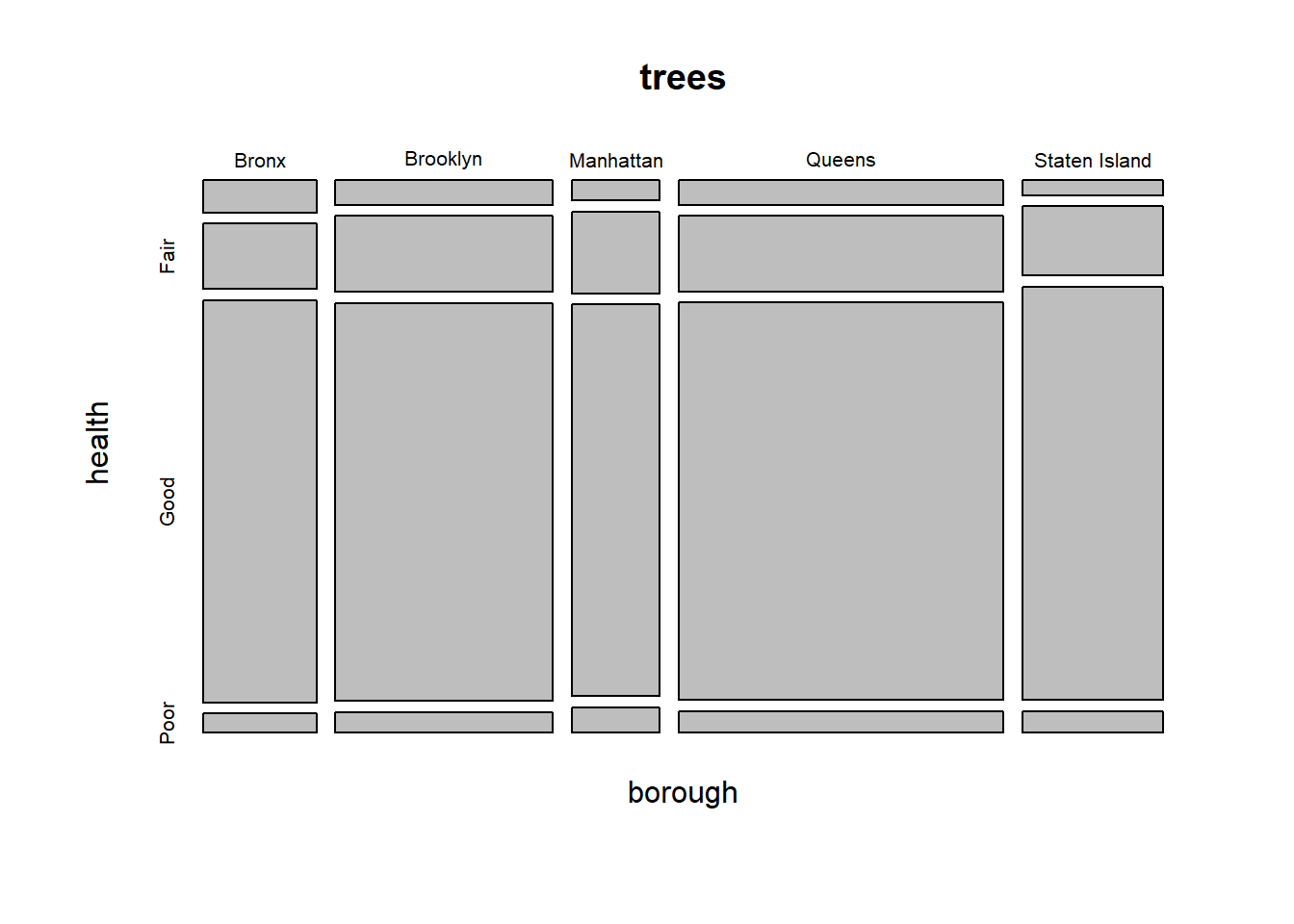## 3.2ggplot2 require(ggplot2) ### 3.2.1 Basics: aesthetics, facets, geoms We can set the data and mapping arguments either in the global ggplot() call or inside each geom: • Setting it in the global call uses those arguments for the entire plot • Setting it within a geom uses those arguments just for that geom, overriding the global options # Set color by class # Just set an aesthetic to name of a variable ggplot(data = mpg) + geom_point(mapping = aes(x = displ, y = hwy, color = class)) + labs( title = "Mileage versus engine size", subtitle = "by class of car", caption = "Courtesy of Hadley Wickham" )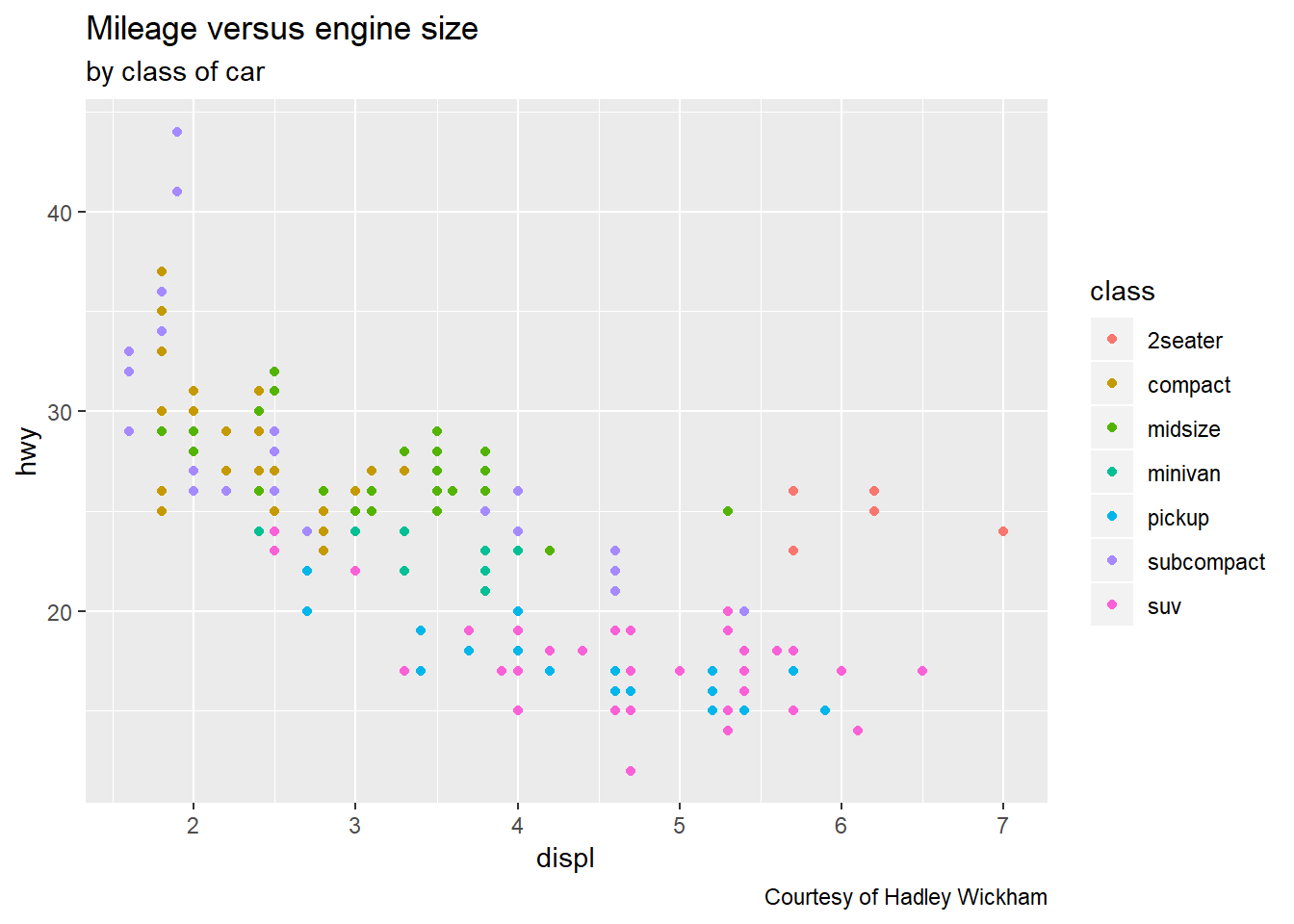# Set an aesthetic property # Use the property outside aes() but inside the geom ggplot(data = mpg) + geom_point(mapping = aes(x = displ, y = hwy), color = "red")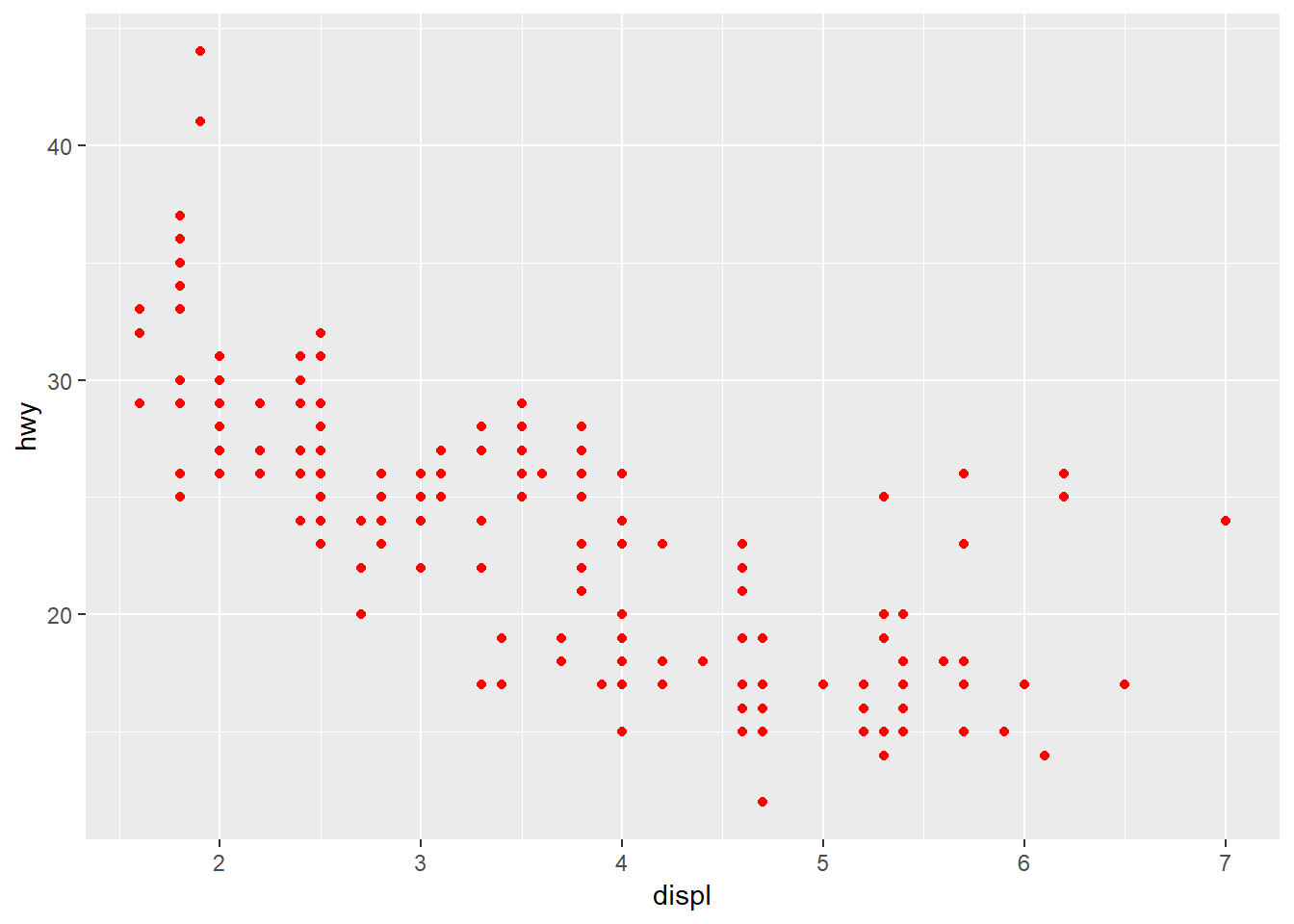To facet, use facet_wrap() or facet_grid(): # Facet by one variable (ggplot will wrap automatically) ggplot(data = mpg) + geom_point(mapping = aes(x = displ, y = hwy)) + facet_wrap(~ class, nrow = 2) # note specification of formula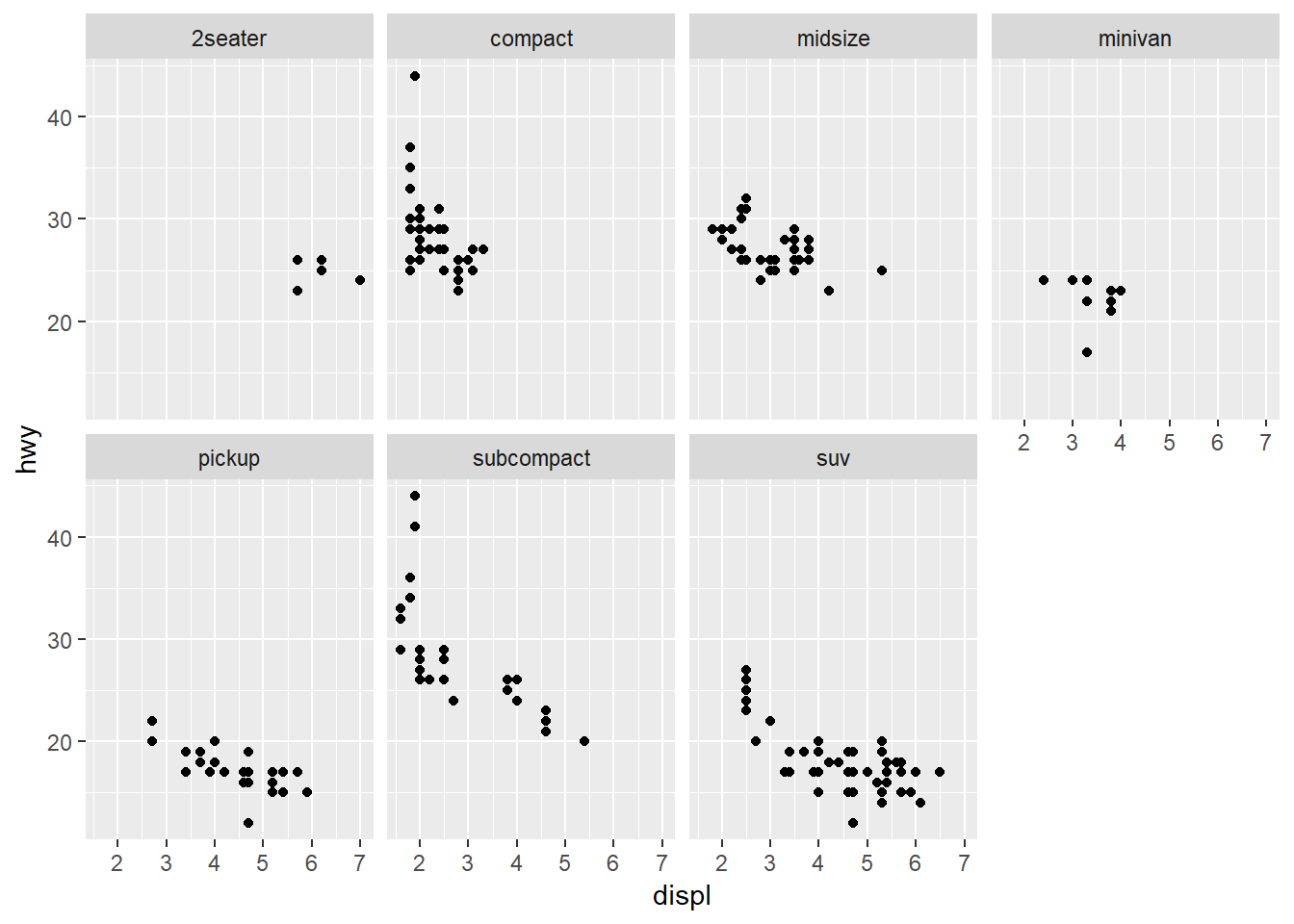# Facet by two variable ggplot(data = mpg) + geom_point(mapping = aes(x = displ, y = hwy)) + facet_grid(drv ~ cyl) # note specification of formula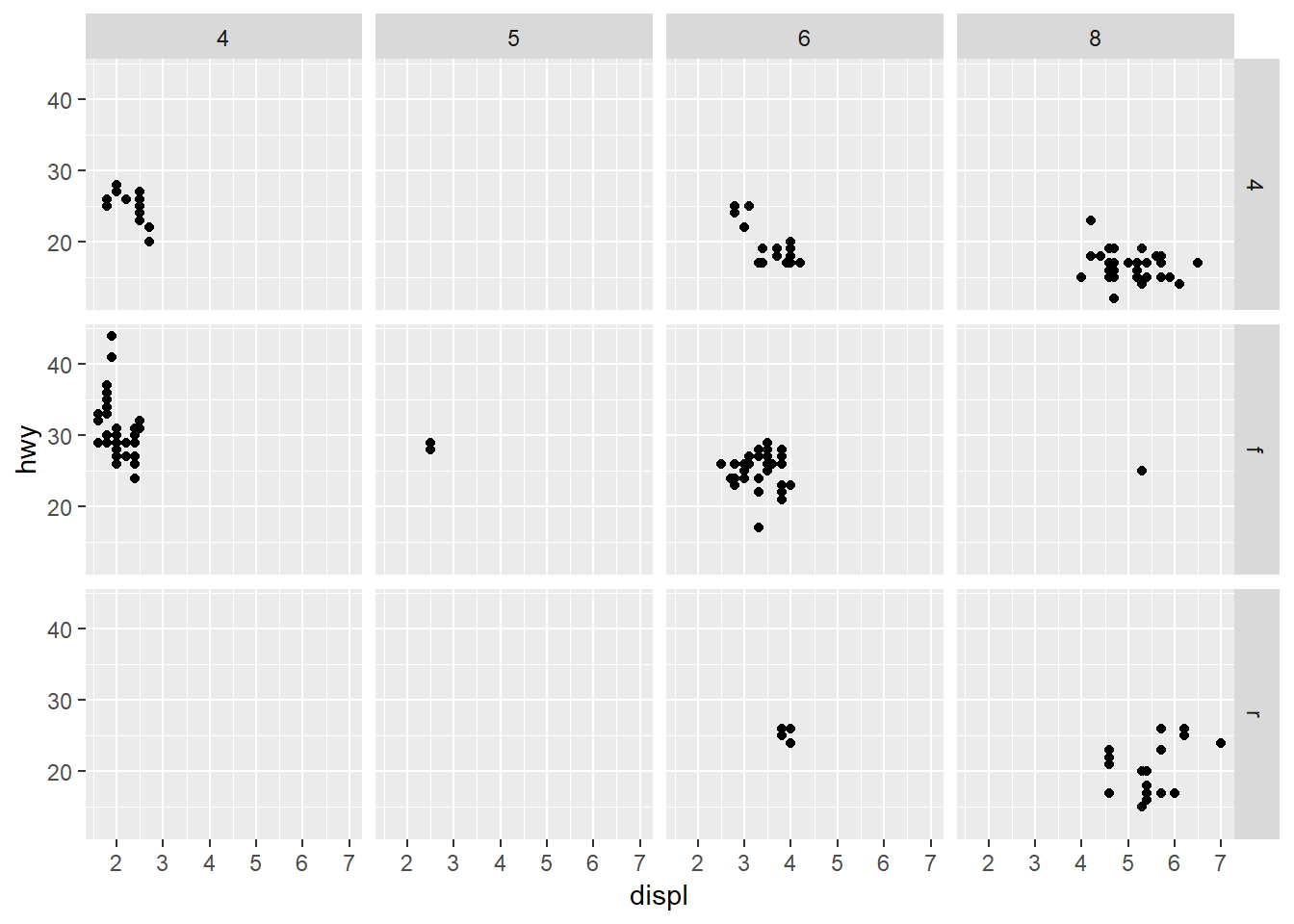Jittering is done with geom_jitter() or with geom_point(position = "jitter"). Set format of bar charts using the position argument in geom_bar(): base <- ggplot(diamonds, aes(x = cut, fill = clarity)) # note that plots can be assigned to variables and constructed additively base + geom_bar(position = "stack") # this is the default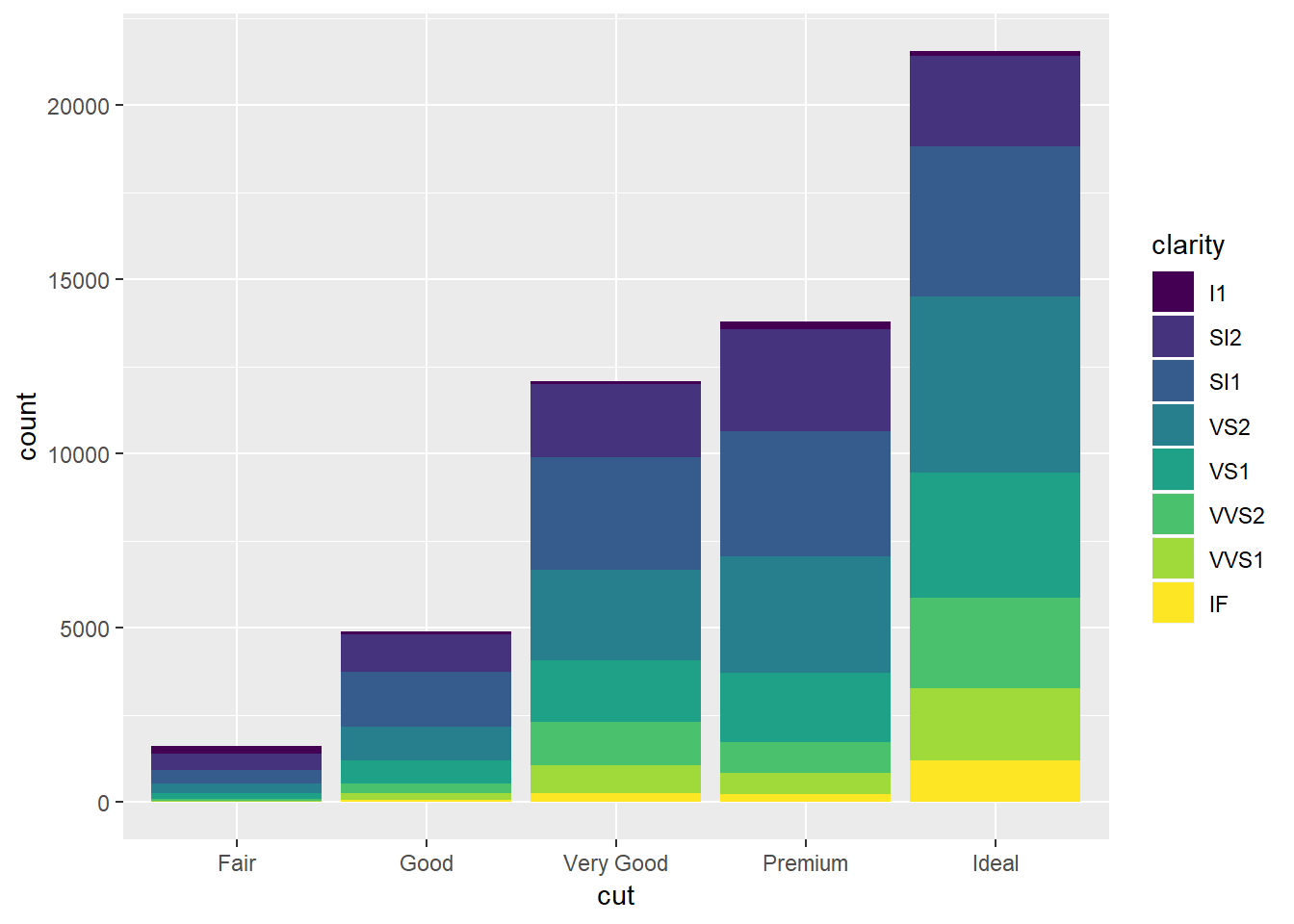base + geom_bar(position = "identity") # bars are overlapping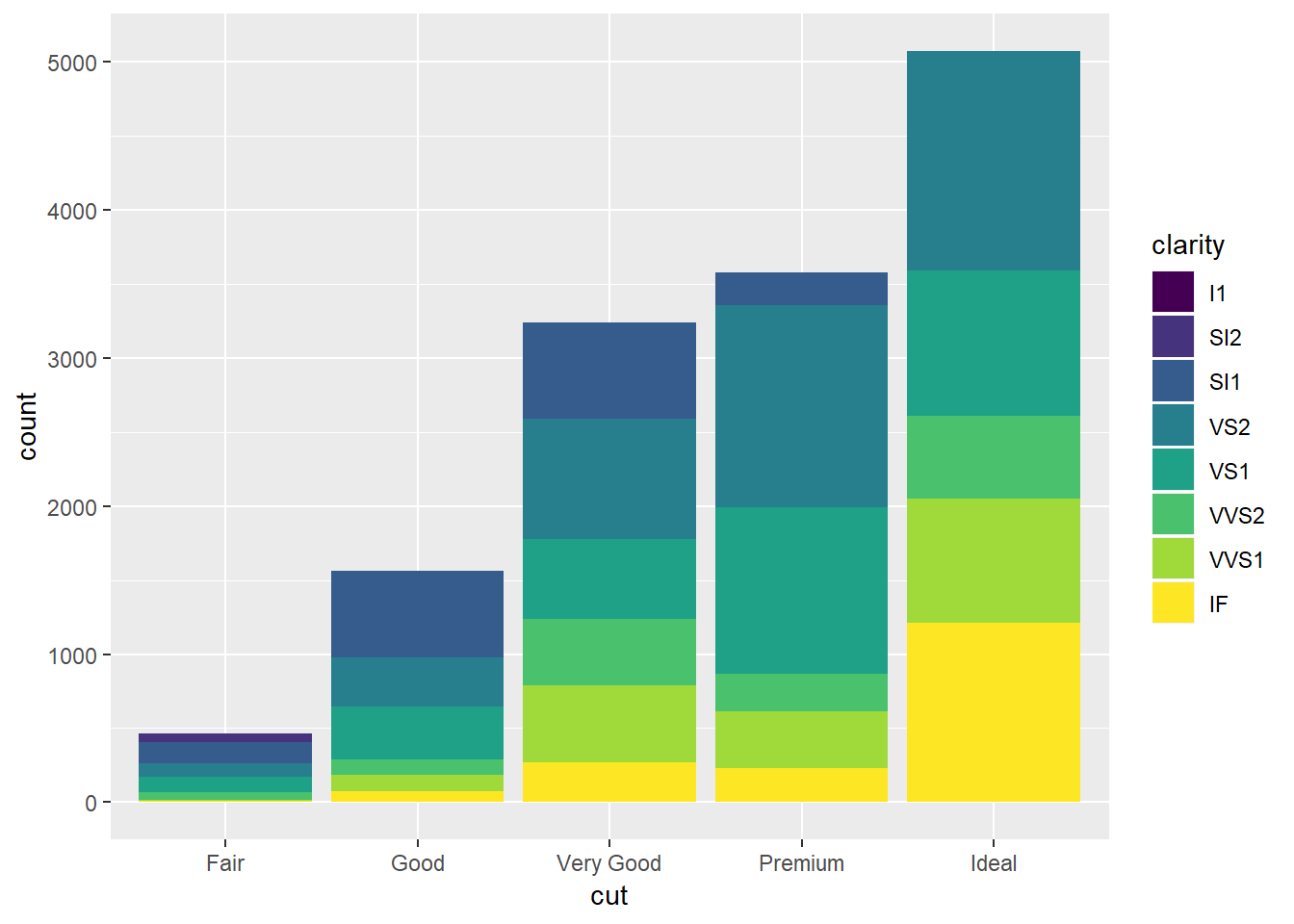base + geom_bar(position = "dodge") # side by side barplot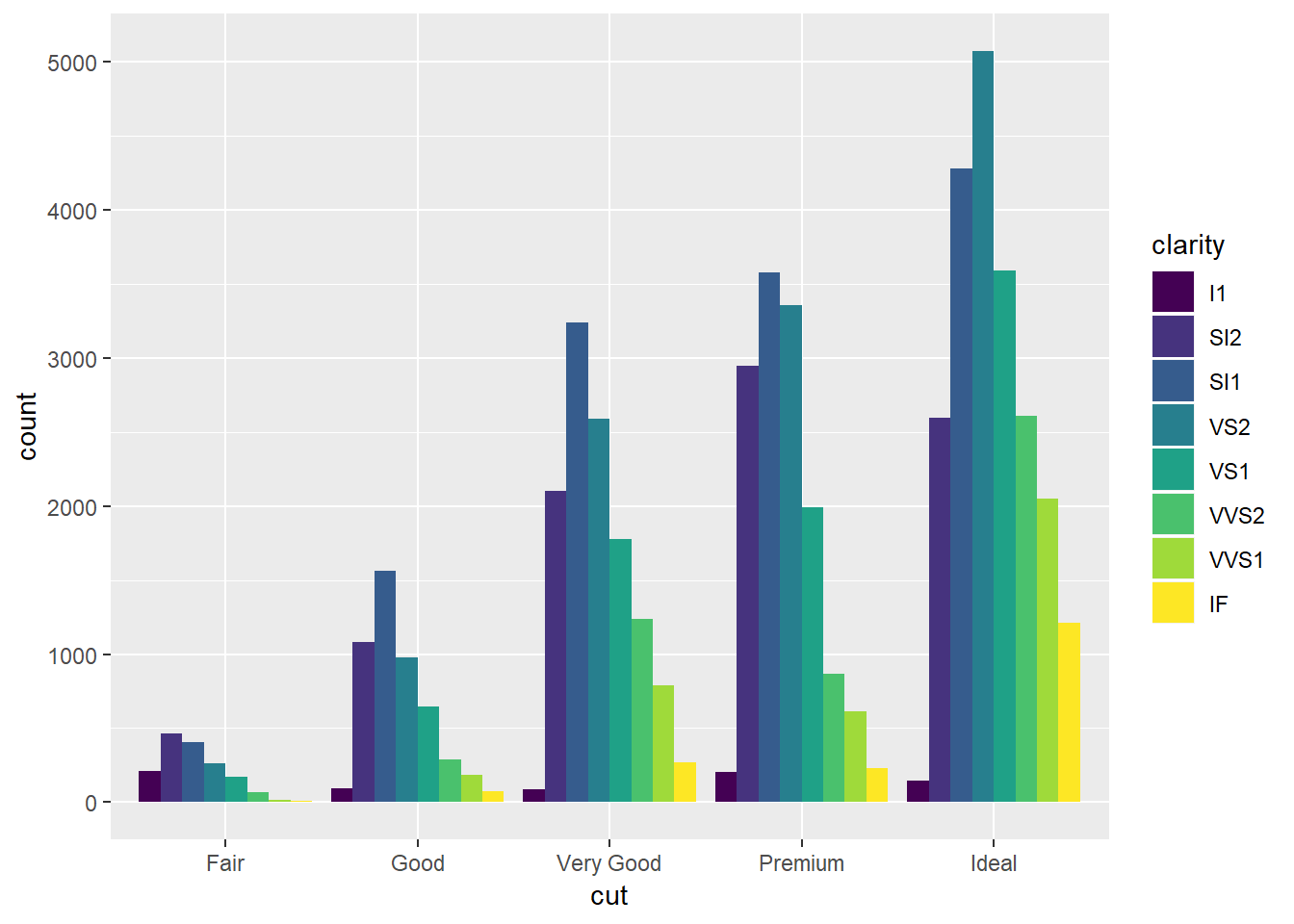base + geom_bar(position = "fill") # proportion within each group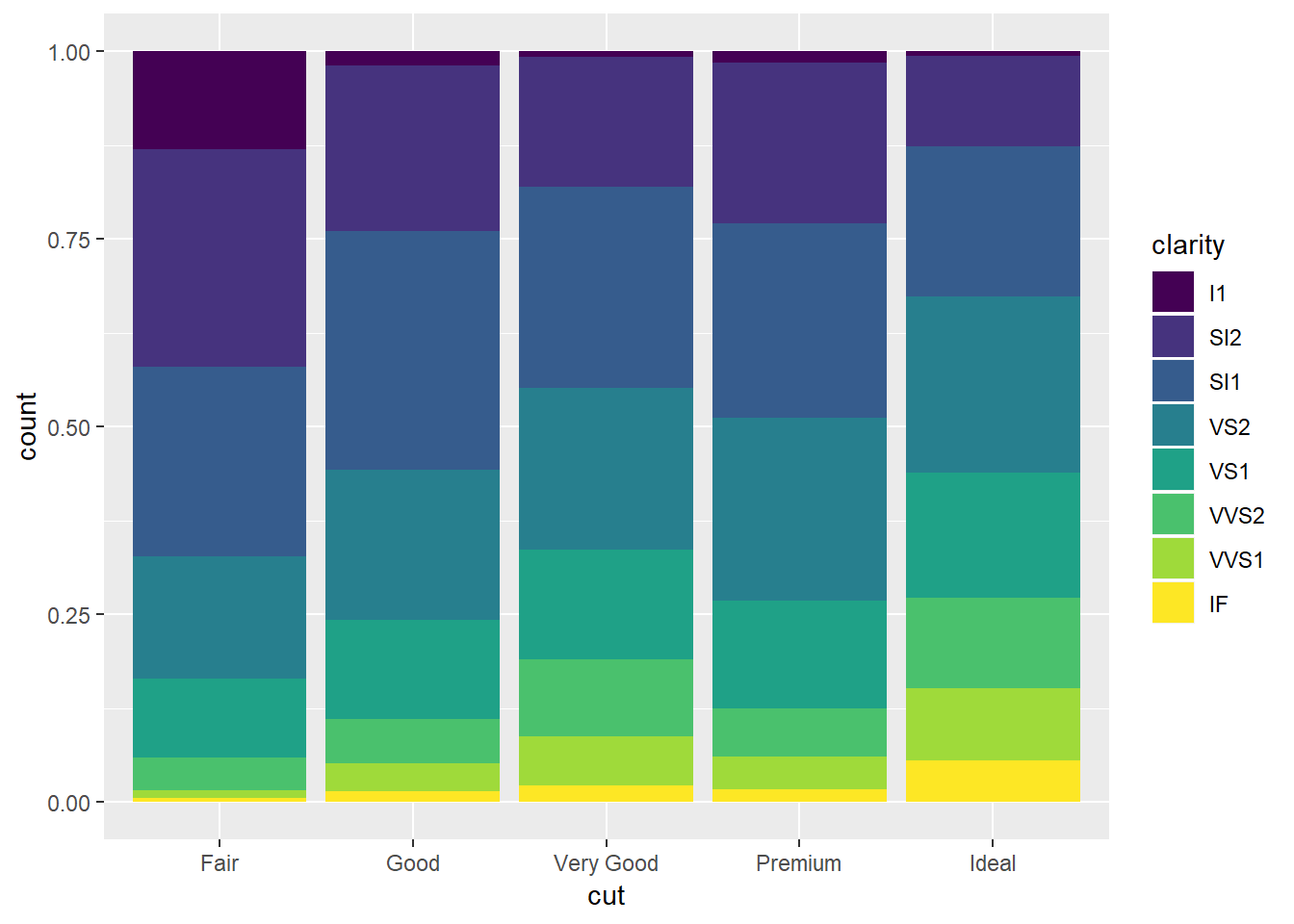To flip what’s plotted on the x-axis versus y-axis, use coord_flip(). ### 3.2.2 Quick plots with qplot qplot provides a wrapper for creating ggplots that: • Is more convenient to use quickly • Has most (but not all) features in day-to-day use • Creates a ggplot object, so can be manipulated (i.e. additively) in the same way Note that unlike plot() from base-R, qplot does not accept formula notation! qplot(displ, hwy, data = mpg, main = "Title here", xlab = "x-axis label") # basic structure qplot(displ, hwy, data = mpg, facets = ~ class) # faceting: use either a one- or two-sided formula qplot(displ, hwy, data = mpg, color = class) # same graph as before, much less code! qplot(displ, hwy, data = mpg, color = I("red")) # surround in I() to set aesthetic qplot(displ, hwy, data = mpg, geom = c("point", "smooth", "jitter")) # multiple geoms (with jitter) qplot(hwy, data = mpg) # only specify x creates histogram qplot(y = hwy, data = mpg, geom = "boxplot") # only specify x creates histogram ### 3.2.3 Fonts and customization ## 3.3 Interactive plots using plotly ## 3.4 Interactive plots using shiny # 4 Statistics ## 4.1 Normality Generally, use the normal quantile plot fom car: require(car) car::qqPlot(trees$tree_dbh)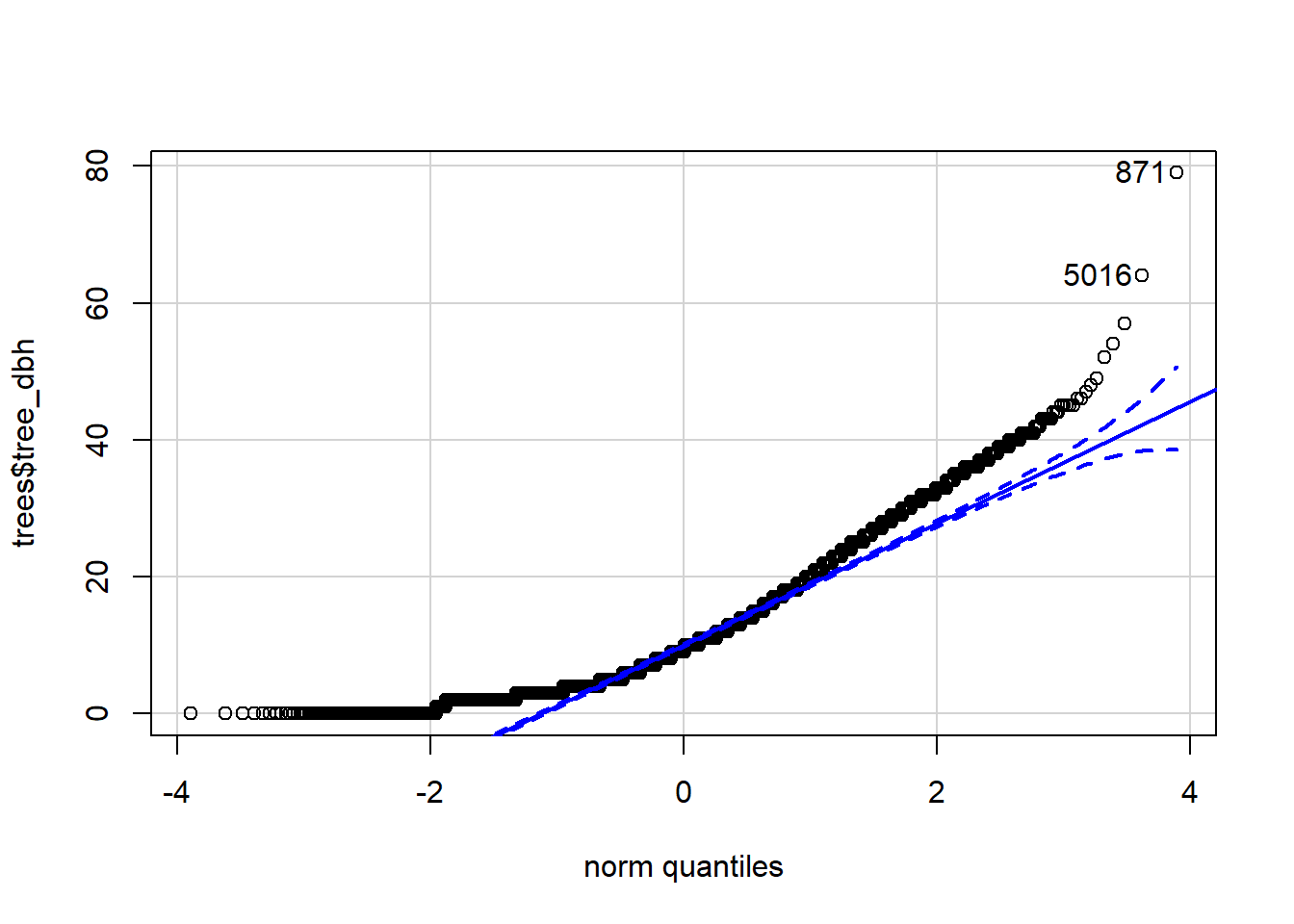##   871 5016

## 4.2 Transformations

### 4.2.1 Box-Cox transformation

Use either one of these functions, both in the car package:

• car::boxCox generates a plot on creation, even when assigning to an object
• car::powerTransform finds per-column Box-Cox powers (and includes several transformation options. car::bcPower raises each column of data to each specified value of lambda.
boxcox <- car::boxCox(name_of_model) # generates plot
power <- boxcox$x[which.max(boxcox$y)]

# For multiple columns at once
bc_param <- car::powerTransform(data)
df_new <- bcPower(data, bc_param$lambda) # bc_param <- car::powerTransform(data, family = "bcPower") -- default # bc_param <- car::powerTransform(data, family = "bcnPower") -- allows negatives ### 4.2.2 Logit transformation ## 4.3 Heteroskedasticity ### 4.3.1 Bartlett’s test for equality of variances Bartlett’s test assumes data come from a normal distribution within each group • In general, a very sensitive test, so use with a grain of salt • Very sensitive to departures from non-normality, so if data is non-normal, Barlett’s test might just be testing for that instead • Note that the null hypothesis is $$H_0: \text{variances are equal}$$, so we actually want to fail to reject the null • We’ve technically only concluded that we don’t know that the variances aren’t different, but in practice we ignore this distinction bartlett.test(trees$tree_dbh, trees$borough) ## ## Bartlett test of homogeneity of variances ## ## data: trees$tree_dbh and trees$borough ## Bartlett's K-squared = 441.91, df = 4, p-value < 2.2e-16 bartlett.test(tree_dbh ~ borough, data = trees) # same thing ## ## Bartlett test of homogeneity of variances ## ## data: tree_dbh by borough ## Bartlett's K-squared = 441.91, df = 4, p-value < 2.2e-16 ### 4.3.2 Levene’s test for equality of variances Levene’s test is similar, but doesn’t assume normality within each group (but has lower power): car::leveneTest(trees$tree_dbh, trees$borough) ## Levene's Test for Homogeneity of Variance (center = median) ## Df F value Pr(>F) ## group 4 90.561 < 2.2e-16 *** ## 9995 ## --- ## Signif. codes: 0 '***' 0.001 '**' 0.01 '*' 0.05 '.' 0.1 ' ' 1 car::leveneTest(tree_dbh ~ borough, data = trees) # same thing ## Levene's Test for Homogeneity of Variance (center = median) ## Df F value Pr(>F) ## group 4 90.561 < 2.2e-16 *** ## 9995 ## --- ## Signif. codes: 0 '***' 0.001 '**' 0.01 '*' 0.05 '.' 0.1 ' ' 1 ### 4.3.3 Equality of covariances for multivariate tests (Box’s M) Compute covariance matrices for each group, or use boxM() from biotools. for(name in levels(sensing$Crop)) { # loop through categories of factor variable
print(cov(sensing[sensing$Crop == name, -1])) } #s: boxM(predictors, response) biotools::boxM(data[, -1], data$Crop)

## 4.4 Basic hypothesis tests

### 4.4.1 Simple t-test for means

# Generate data
n <- 100
rand <- data.frame(id = 1:n,
group = sample(1:2, 100, replace = TRUE),
var1 = runif(n, -10, 10),
var2 = runif(n, -8, 12))
head(rand)
##   id group       var1       var2
## 1  1     1 -5.2025253  4.0520755
## 2  2     1 -0.3507774 -4.6487709
## 3  3     2  4.5811925  9.7107064
## 4  4     2 -0.4222238  9.4746105
## 5  5     1 -2.8241554 10.4507917
## 6  6     1  0.9294305 -0.9094015
# One-sample t-test
t.test(rand$var1) # tests if equal to 0 ## ## One Sample t-test ## ## data: rand$var1
## t = -0.36117, df = 99, p-value = 0.7187
## alternative hypothesis: true mean is not equal to 0
## 95 percent confidence interval:
##  -1.3157912  0.9105532
## sample estimates:
## mean of x
## -0.202619
t.test(rand$var1, mu = 2, conf.level = 0.99) # tests if equal to 2 ## ## One Sample t-test ## ## data: rand$var1
## t = -3.9261, df = 99, p-value = 0.0001596
## alternative hypothesis: true mean is not equal to 2
## 99 percent confidence interval:
##  -1.676068  1.270830
## sample estimates:
## mean of x
## -0.202619
# Two-sample t-test, paired
t.test(rand$var1, rand$var2, paired = TRUE)
##
##  Paired t-test
##
## data:  rand$var1 and rand$var2
## t = -2.1521, df = 99, p-value = 0.03382
## alternative hypothesis: true difference in means is not equal to 0
## 95 percent confidence interval:
##  -3.4609316 -0.1404602
## sample estimates:
## mean of the differences
##               -1.800696
# Two-sample t-test, unpaired
t.test(var1 ~ group, data = rand)
##
##  Welch Two Sample t-test
##
## data:  var1 by group
## t = 1.6455, df = 96.646, p-value = 0.1031
## alternative hypothesis: true difference in means is not equal to 0
## 95 percent confidence interval:
##  -0.3764253  4.0268413
## sample estimates:
## mean in group 1 mean in group 2
##       0.7282371      -1.0969709

## 4.7 Cross-validation

Base R sample() function syntax: sample(from, n, replace = FALSE)

# 5 Generalized linear models

## 5.1 Multiple regression

### 5.1.1 Creating the model

# Create basic model using lm
wb_lm <- lm(Exports ~ Imports, data = wb)

# Plot with regression line
par(mar = c(5.1, 4.1, 4.1, 2.1)) # default R settings
plot(Exports ~ Imports, data = wb, col = "red", pch = 19)

### 5.1.3 Robust standard error

After creating a linear model using standard base R lm, use:

lmtest::coeftest(lm, vcov = sandwich::vcovHC(lm, type = "HC1"))

Note: default for type is “HC3”, but must set to “HC1” to obtain same results as Stata

TODO: look more into the rlm() functions in MASS

### 5.1.4 Joint hypothesis tests

After creating a linear model, use car::linearHypothesis():

car::linearHypothesis(lm, c("meduc = 0", "feduc = 0")) # homoskedastic only
car::linearHypothesis(lm, c("meduc = 0", "feduc = 0"), white.adjust = "hc1") # robust

TODO: look more into options in car::linearHypothesis()

## 5.2 Analysis of variance

### 5.2.1 ANOVA-regression equivalence

Since ANOVA is essentially the same as linear regression with categorical predictors, many of the methods are similar:

trees_anova <- aov(tree_dbh ~ borough, data = trees)
summary(trees_anova)
##               Df Sum Sq Mean Sq F value Pr(>F)
## borough        4  20771    5193   72.72 <2e-16 ***
## Residuals   9995 713742      71
## ---
## Signif. codes:  0 '***' 0.001 '**' 0.01 '*' 0.05 '.' 0.1 ' ' 1
summary.lm(trees_anova)
##
## Call:
## aov(formula = tree_dbh ~ borough, data = trees)
##
## Residuals:
##     Min      1Q  Median      3Q     Max
## -12.709  -6.495  -1.709   4.513  67.103
##
## Coefficients:
##                      Estimate Std. Error t value Pr(>|t|)
## (Intercept)            9.4617     0.2362  40.059  < 2e-16 ***
## boroughBrooklyn        2.4348     0.2911   8.363  < 2e-16 ***
## boroughManhattan      -0.9663     0.3578  -2.701  0.00693 **
## boroughQueens          3.2474     0.2742  11.842  < 2e-16 ***
## boroughStaten Island   1.2648     0.3175   3.984 6.82e-05 ***
## ---
## Signif. codes:  0 '***' 0.001 '**' 0.01 '*' 0.05 '.' 0.1 ' ' 1
##
## Residual standard error: 8.45 on 9995 degrees of freedom
## Multiple R-squared:  0.02828,    Adjusted R-squared:  0.02789
## F-statistic: 72.72 on 4 and 9995 DF,  p-value: < 2.2e-16
trees_lm <- lm(tree_dbh ~ borough, data = trees)
summary(trees_lm)
##
## Call:
## lm(formula = tree_dbh ~ borough, data = trees)
##
## Residuals:
##     Min      1Q  Median      3Q     Max
## -12.709  -6.495  -1.709   4.513  67.103
##
## Coefficients:
##                      Estimate Std. Error t value Pr(>|t|)
## (Intercept)            9.4617     0.2362  40.059  < 2e-16 ***
## boroughBrooklyn        2.4348     0.2911   8.363  < 2e-16 ***
## boroughManhattan      -0.9663     0.3578  -2.701  0.00693 **
## boroughQueens          3.2474     0.2742  11.842  < 2e-16 ***
## boroughStaten Island   1.2648     0.3175   3.984 6.82e-05 ***
## ---
## Signif. codes:  0 '***' 0.001 '**' 0.01 '*' 0.05 '.' 0.1 ' ' 1
##
## Residual standard error: 8.45 on 9995 degrees of freedom
## Multiple R-squared:  0.02828,    Adjusted R-squared:  0.02789
## F-statistic: 72.72 on 4 and 9995 DF,  p-value: < 2.2e-16
summary.aov(trees_lm) 
##               Df Sum Sq Mean Sq F value Pr(>F)
## borough        4  20771    5193   72.72 <2e-16 ***
## Residuals   9995 713742      71
## ---
## Signif. codes:  0 '***' 0.001 '**' 0.01 '*' 0.05 '.' 0.1 ' ' 1
• By default, calling summary() on an aov object calls summary.aov() (which prints the usual ANOVA-style sum of squares output), but we can also force R to print regression-like output for an ANOVA model by calling summary.lm() on an aov object
• Can do the same for lm by calling summary.aov() on an lm object

### 5.2.2 Confidence intervals for group means

We can actually tell R to fit a regression model where the coefficient estimates are the group means by fitting a model without an intercept, which we do by adding -1 to the regression specification:

• We can do this when we have only one categorical predictor since the base level essentially “replaces” the intercept, using up the degree of freedom that the intercept previously used
• Then, the coef() function returns the means, and confint() returns confidence intervals
trees_lm2 <- lm(tree_dbh ~ borough - 1, data = trees)
coef(trees_lm2) # same as tapply(trees$tree_dbh, trees$borough, mean)
##         boroughBronx      boroughBrooklyn     boroughManhattan
##             9.461719            11.896552             8.495450
##        boroughQueens boroughStaten Island
##            12.709160            10.726528
round(confint(trees_lm2), 2) # 95% CI for individual group means
##                      2.5 % 97.5 %
## boroughBronx          9.00   9.92
## boroughBrooklyn      11.56  12.23
## boroughManhattan      7.97   9.02
## boroughQueens        12.44  12.98
## boroughStaten Island 10.31  11.14

plotCI() from plotrix can help in plotting confidence intervals:

trees_means <- coef(trees_lm2)
trees_ci <- confint(trees_lm2)

par(mar = c(5, 8, 4, 2))
plotrix::plotCI(x = trees_means, # x-values (coef estimates)
y = 1:length(trees_means), # y-values (just 1 through 5)
ui = trees_ci[, 2], # upper bound of each error bar
li = trees_ci[, 1], # lower bound of each error bar
err = "x", # direction of bars (x is horizontal)
xlab = "tree dbh", ylab = "", main = "Means and CIs for tree height",
axes = FALSE, lwd = 2, col = "blue")
axis(side = 1)
axis(side = 2, at = 1:(length(trees_means)), label = levels(trees\$borough), las = 2)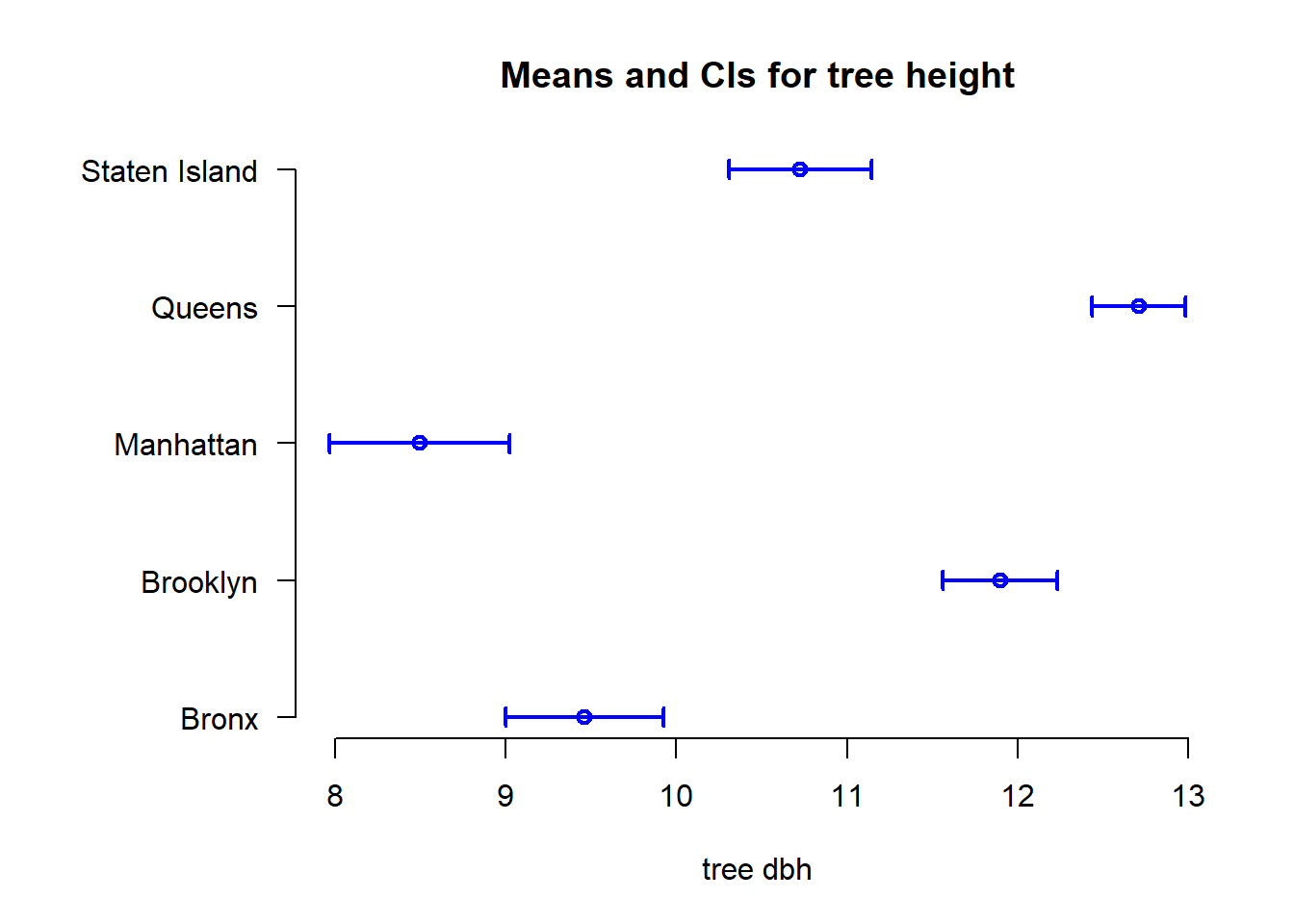### 5.2.3 Multiple comparisons

Best way to perform multiple comparisons is using Tukey HSD:

trees_tukey <- TukeyHSD(trees_anova, conf.level = 0.95)

par(mar = c(5, 11, 4, 1))
plot(trees_tukey, las = 1)